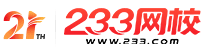#计算机二级

•扫描二维码进入

• 题 库扫描二维码进入

• 移动233网校移动

• 移动APP扫描二维码下载APP

## 2019年3月计算机二级C语言考试选择题强化练习014

(1)以下叙述中错误的是( )。

A.C程序在运行过程中所有计算都以二进制方式进行

B.C程序在运行过程中所有计算都以十进制方式进行

C.所有C程序都需要编译链接无误后才能运行

D.C程序中字符变量存放的是字符的ASCIl码值

(2)以下关于C语言的叙述中正确的是(　　)。

A.C语言的数值常量中夹带空格不影响常量值的正确表示

B.c语言中的变量可以在使用之前的任何位置进行定义

C.在c语言算术表达式的书写中，运算符两侧的运算数类型必须一致

D.C语言中的注释不可以夹在变量名或关键字的中间

(3)以下不合法的字符常量是(　　)。

A.'\\'

B.'\"'

C.'\018'

D.'\xcc'

(4)以下选项中正确的定义语句是(　　)。

A.double，a，b;

B.double a=b=7;

C.double a;b;

D.double a=7，b=7;

(5)若有定义语句：int a=3，b=2，c=1;以下选项中错误的赋值表达式是(　　)。

A.a=(b=4)=3;

B.a=b=c+1;

C.a=(b=4)+c;

D.a=1+(b=c=4);

(6)若有定义：int a，b;通过语句：scanf("%d;%d"，&a，&B.;能把整数3赋给变量a，5赋给变量b的输入数据是(　　)。

A.3 5

B.3，5

C.3;5

D.35

(7)以下不合法的数值常量是(　　)。

A.8.0E0.5

B.1e1

C.011

D.Oxabcd

(8)以下关于C语言数据类型使用的叙述中错误的是(　　)。

A.若要处理如“人员信息”等含有不同类型的相关数据，应自定义结构体类型

B.若要保存带有多位小数的数据，可使用双精度类型

C.若只处理“真”和“假”两种逻辑值，应使用逻辑类型

D.整数类型表示的自然数是准确无误差的

(9)设有定义：int k=0;以下选项的四个表达式中与其他三个表达式的值不相同的是(　　)。

A.++k

B.k+=1

C.k++

D.k+1

(10)有如下程序段：

int x=12;

double y=3.141593;

prinff("%d%8.6f"，x，y);其输出结果是( )。

A.123.141593

B.12 3.141593

C.12，3.141593

D.123.1415930

• 海量免费模拟试题，在线测试。

• 各科每年真题试卷，实战演练！

• 逐章逐节针对练习，专项突破。

• 每天10道最新试题，随机练习。

• QQ群号：806931213

扫描二维码加群• ##### 微信订阅号：kszx233

扫描二维码加群